# ISNA function and how to use it to ignore the error in Vlookup formula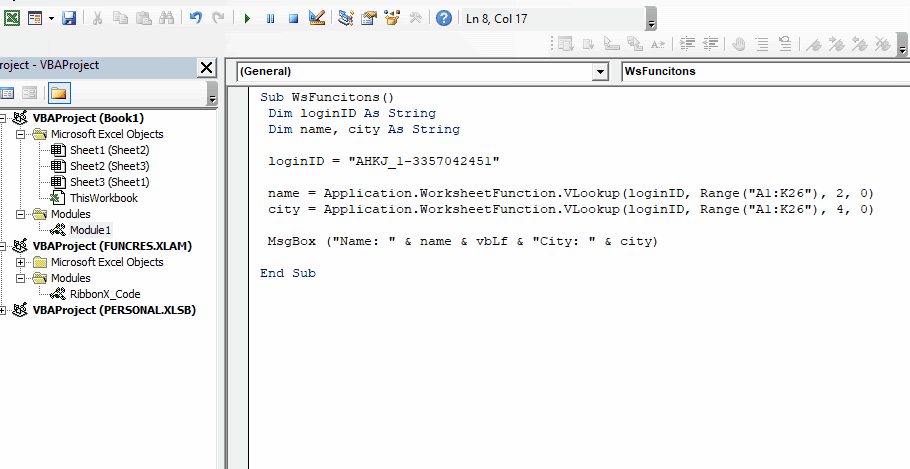The functions like VLOOKUP, COUNTIF, SUMIF are called worksheet functions. Generally, the functions that are predefined in Excel and ready to use on a worksheet are worksheet functions. You can't alter or see the code behind these functions in VBA.

On the other hand the user defined functions and functions specific to VBA like MsgBox or InputBox are VBA functions.

We all know how to use VBA functions in VBA. But what if we want to use VLOOKUP in a VBA. How do we do that? In this article, we will  explore exactly that.

#### Using Worksheet Functions in VBA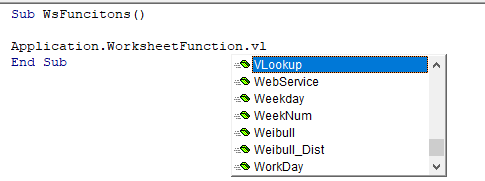To access the worksheet function we use an Application class. Almost all the worksheet functions are listed into Application.Worksheet Function class. And using dot operator, you can access them all.

In any sub, write Application.Worksheet Function. And start writing the name of the function. VBA's intellisense will show the name of the functions available to use. Once you have chosen the function name, it will ask for the variables, like any function on excel. But you will have to pass variables in VBA understandable format. For example, if you want to pass a range A1:A10, you will have to pass it as a range object like Range("A1:A10").

So let's use some worksheet functions to understand it better.

#### How to Use VLOOKUP function in VBA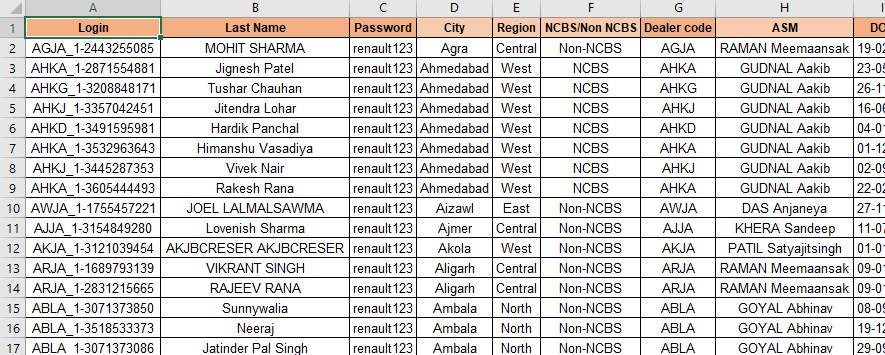To demonstrate how you can use a VLOOKUP function in VBA, here I have sample data. I need to show the Name and City of given Login Id in a message box using VBA. The data is spread in range A1:K26.

Press ALT+F11 to open VBE and insert a module.

See the below code.

```Sub WsFuncitons()

Dim loginID As String
Dim name, city As String

'Using VLOOKUP function to get name of given id in table
name = Application.WorksheetFunction.VLookup(loginID, Range("A1:K26"), 2, 0)

'Using VLOOKUP function to get city of given id in table
city = Application.WorksheetFunction.VLookup(loginID, Range("A1:K26"), 4, 0)

MsgBox ("Name: " & name & vbLf & "City: " & city)

End Sub
```

When you run this code, you will get this result.You can see how quickly the VBA prints the result into a message box.  Now let's examine the code.

How does it work?

1.

Dim loginID As String

Dim name, city As String

First we have declared two variables of string type to store the result returned by the VLOOKUP function. I have used string type variables because I am sure that the result returned by VLOOKUP will be a string value. If your worksheet function is expected to return number, date, range, etc. type of value, use that kind of variable to store the result. If you are not sure, which kind of value will be returned by the worksheet function, use variant type variables.

2.

Next we have used the loginID variable to store lookup value. Here we have used a hardcoded value. You can use references too. For example. You can use Range("A2").Value to dynamically update lookup value from range A2.

3.

name = Application.WorksheetFunction.VLookup(loginID, Range("A1:K26"), 2, 0)

Here we use the VLOOKUP function to get. Now when you right the function and open the parenthesis, it will show you arguments required but not as descriptive as it shows on the Excel. See for yourself.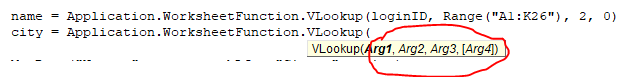You need to remember how and what variable you need to use. You can always go back to the worksheet to see the descriptive variable details.

Here, the lookup value is Arg1. For Arg1 we use loginID. The lookup table is Arg2. For Arg2 we used Range("A1:K26"). Note that we did not use directly A2:K26 as we do on the Excel. The columns index is Arg3. For Arg3 we used 2, since the name is in the second column. The lookup type is Arg4. We used 0 as Arg4.

city = Application.WorksheetFunction.VLookup(loginID, Range("A1:K26"), 4, 0)

Similarly we get the city name.

4.

MsgBox ("Name: " & name & vbLf & "City: " & city)

Finally we print name and city using Messagebox.

#### Why use Worksheet Function in VBA?

The worksheet functions possess power of immense calculations and it won't be smart to ignore the power of worksheet functions. For example, if we want the standard deviation of a dataset and you want to write whole code for this, it can take you hours. But if you know how to use the worksheet function STDEV.P in VBA to get the calculation in one go.

```Sub GetStdDev()

std = Application.WorksheetFunction.StDev_P(Range("A1:K26"))

End Sub
```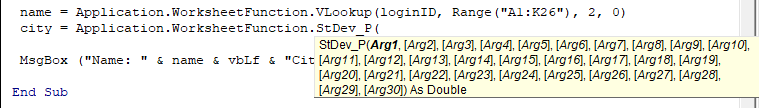Using Multiple Worksheet Functions VBA

Let's say we need to use an index match to retrieve some values. Now how would you wright the formula in VBA. This is what I guess you will write:

```Sub IndMtch()

Val = Application.WorksheetFunction.Index(result_range, _
Application.WorksheetFunction.Match(lookup_value, _
lookup_range, match_type))

End Sub

```

This is not wrong but it is lengthy. The right way to use multiple functions is by using a With block. See the below example:

```Sub IndMtch()

With Application.WorksheetFunction
Val = .Index(result_range, .Match(lookup_value, lookup_range, match_type))
val2 = .VLookup(arg1, arg2, arg3)
val4 = .StDev_P(numbers)
End With
End Sub

```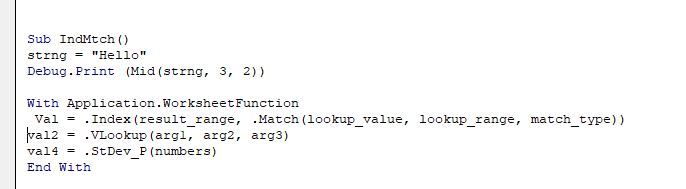As you can see, I have used With block to tell VBA that I will be using the properties and functions of Application.WorksheetFunction. So I do not need to define it everywhere. I just used the dot operator to access INDEX, MATCH, VLOOKUP and STDEV.P functions. Once we use End With statement, we are not able to access functions without using fully qualified function names.

So if you have to use multiple worksheet functions in VBA, use with block.

Not All the Worksheet Function are Available Through Application.WorksheetFunction

Some Worksheet Functions are directly available to use in VBA. You don't need to use Application.WorksheetFunction object.

For example, functions like Len() which is used to get the number of characters in a string, left, right, mid, trim, offset etc. These functions can be directly used in VBA. Here is an example.

```Sub GetLen()
Strng = "Hello"
Debug.Print (Len(strng))
End Sub

```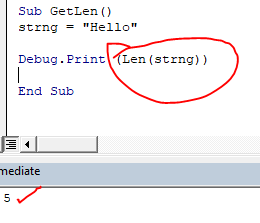See, here we used the LEN function without using Application.WorksheetFunction object.

Similarly you can use other functions like left, right, mid, char etc.

```Sub GetLen()

Strng = "Hello"
Debug.Print (Len(strng))
Debug.Print (left(strng,2))
Debug.Print (right(strng,1))
Debug.Print (Mid(strng, 3, 2))

End Sub
When you run the above sub, it will return:```
 5 He o ll

Hope this article about How to Use Worksheet Functions like VLOOKUP in VBA in Excel is explanatory. Find more articles on VBA formulas and related Excel formulas here. If you liked our blogs, share it with your friends on Facebook. And also you can follow us on Twitter and Facebook. We would love to hear from you, do let us know how we can improve, complement or innovate our work and make it better for you. Write to us at info@exceltip.com.

Related Articles:

What is CSng Function in Excel VBA : The SCng function is a VBA function that converts any data type to a single-precision floating-point number ("given that it is a number"). I mostly use CSng function to convert text formatted numbers into actual numbers.

How to get Text & Number in Reverse through VBA in Microsoft Excel : To reverse number and text we use loops and mid function in VBA. 1234 will be converted to 4321, "you" will be converted to "uoy". Here's the snippet.

Format data with custom number formats using VBA in Microsoft Excel : To change the number format of specific columns in excel use this VBA snippet. It coverts the number format of specified to specified format in one click.

Using Worksheet Change Event To Run Macro When any Change is Made : So to run your macro whenever the sheet updates, we use the Worksheet Events of VBA.

Run Macro If Any Change Made on Sheet in Specified Range : To run your macro code when the value in a specified range changes, use this VBA code. It detects any change made in the specified range and will fire the event.

Simplest VBA Code to Highlight Current Row and Column Using : Use this small VBA snippet to highlight the current row and column of the sheet.

Popular Articles :

How to use the IF Function in Excel : The IF statement in Excel checks the condition and returns a specific value if the condition is TRUE or returns another specific value if FALSE.

How to use the VLOOKUP Function in Excel : This is one of the most used and popular functions of excel that is used to lookup value from different ranges and sheets.

How to Use SUMIF Function in Excel : This is another dashboard essential function. This helps you sum up values on specific conditions.

How to use the COUNTIF Function in Excel : Count values with conditions using this amazing function. You don't need to filter your data to count specific values. Countif function is essential to prepare your dashboard.

1.